Computer Science

# More with images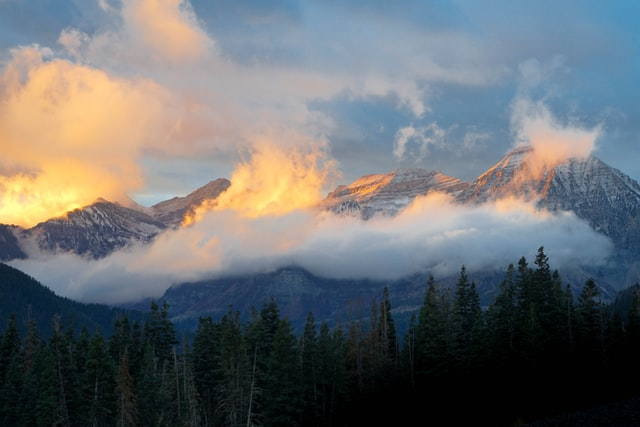## Using range()

### range(n)

• generates a sequence of numbers
• starts from 0
• counts up to and not including n
``````for number in range(10):
print(number)
``````
``````    0
1
2
3
4
5
6
7
8
9``````

### Nested for loops!

``````for y in range(10):
for x in range(5):``````
``````    0 0
1 0
2 0
3 0
4 0
0 1
1 1
...
1 9
2 9
3 9
4 9``````

## Accessing pixels

• uses an x,y coordinate scheme, (0,0) at upper left
• x values move from left to right
• y values move from top to bottom
• each pixel is one color, a mixture of three RGB values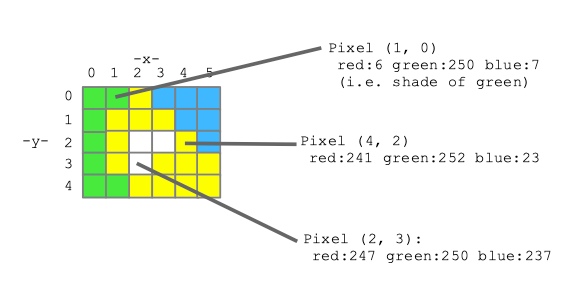### New image functions

• `image.height`: height of the image in pixels
• `image.width`: width of the image in pixels
• `image.get_pixel(x,y)`: get the pixel at location (x,y)
``````from byuimage import Image

image = Image("mount-timpanogos.jpeg")
print(image.height)
print(image.width)
print(f"Height: {image.height}")
print(f"Width: {image.width}")``````
``````    427
640
Height: 427
Width: 640``````

### Let’s loop over the pixels a different way

• nested for loops!
• y = 0, then x = 0, 1, 2, 3, 4
• y = 1, then x = 0, 1, 2, 3, 4

### Let’s loop over the pixels a different way

• nested for loops!
``````from byuimage import Image
def red_channel(filename):
image = Image(filename)
for y in range(image.height):
for x in range(image.width):
pixel = image.get_pixel(x,y)
pixel.green = 0
pixel.blue = 0

return image

red_flower = red_channel("mount-timpanogos.jpeg")
red_flower.show()``````

### Things to try:

• add a print statement inside the nested loops to examine the values of x,y
• try range(image.width - 20)
• try swapping the for loops so we loop on x first, then y

### Make an image darker

``````from byuimage import Image

def darker(image):
""" Pass in an image, modify it """
for y in range(image.height):
for x in range(image.width):
pixel = image.get_pixel(x, y)
pixel.red = pixel.red * 0.5
pixel.green = pixel.green * 0.5
pixel.blue = pixel.blue * 0.5

image = Image("mount-timpanogos.jpeg")
darker(image)
image.show()``````

### What if we don’t want to modify the original?

``````from byuimage import Image

def darker(image):
""" Pass in an image, modify it """
for y in range(image.height):
for x in range(image.width):
pixel = image.get_pixel(x, y)
pixel.red = pixel.red * 0.5
pixel.green = pixel.green * 0.5
pixel.blue = pixel.blue * 0.5

image = Image("mount-timpanogos.jpeg")
darker(image)
image.show()``````

## Making new images

### Copying pixels

• Imagine you have two pixels, a and b
• How can you copy a so that b is the same?
``````b.red = a.red
b.green = a.green
b.blue = a.blue``````

### Create a new, blank image

``````image = Image("mount-timpanogos.jpeg")

# create a blank white 100 x 50 image, store in variable named "new_image"
new_image = Image.blank(100, 50)

# create a blank image the same size as the original
copy_image = Image.blank(image.width, image.height)

# create an image twice as wide as the original
wide_image = Image.blank(image.width * 2, image.height)
wide_image.show()``````

### Copy and make image darker

``````def darker(image):
# Create out image, same size as original
out =  Image.blank(image.width, image.height)
for y in range(image.height):
for x in range(image.width):
pixel = image.get_pixel(x, y)
pixel_out = out.get_pixel(x, y)
pixel_out.red = pixel.red * 0.5
pixel_out.green = pixel.green * 0.5
pixel_out.blue = pixel.blue * 0.5
return out

original = Image("mount-timpanogos.jpeg")

darker_image = darker(original)
darker_image.show()

original.show()``````

## Aqua Stripe Problem

Create an image that has a 100 pixel wide aqua stripe on the left side, with a copy of the image next to it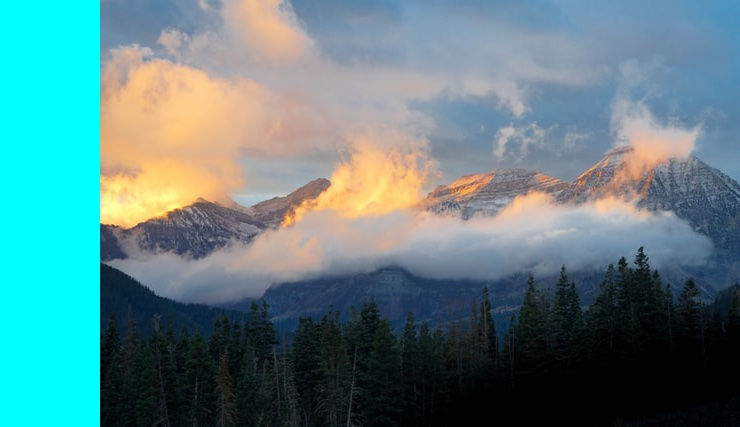### Planning — How big is the image?

``````original = Image("mount-timpanogos.jpeg")
print(f"Height: {original.height}, Width: {original.width}")
``````
``    Height: 427, Width: 640``

### Planning — What do we want?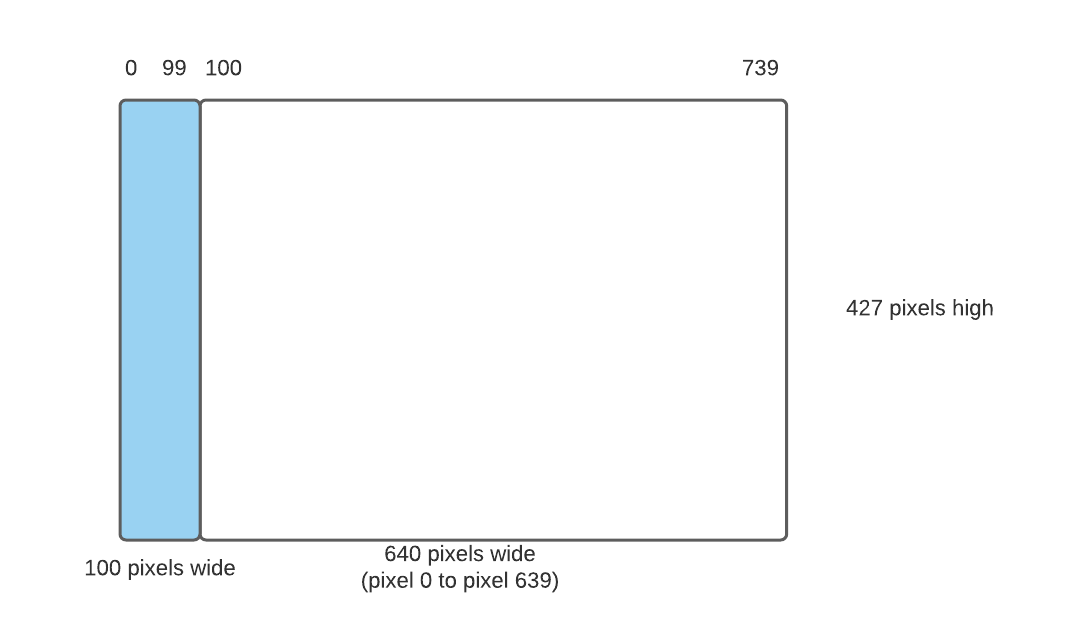### Planning — What do we need to do?

(1) create a new image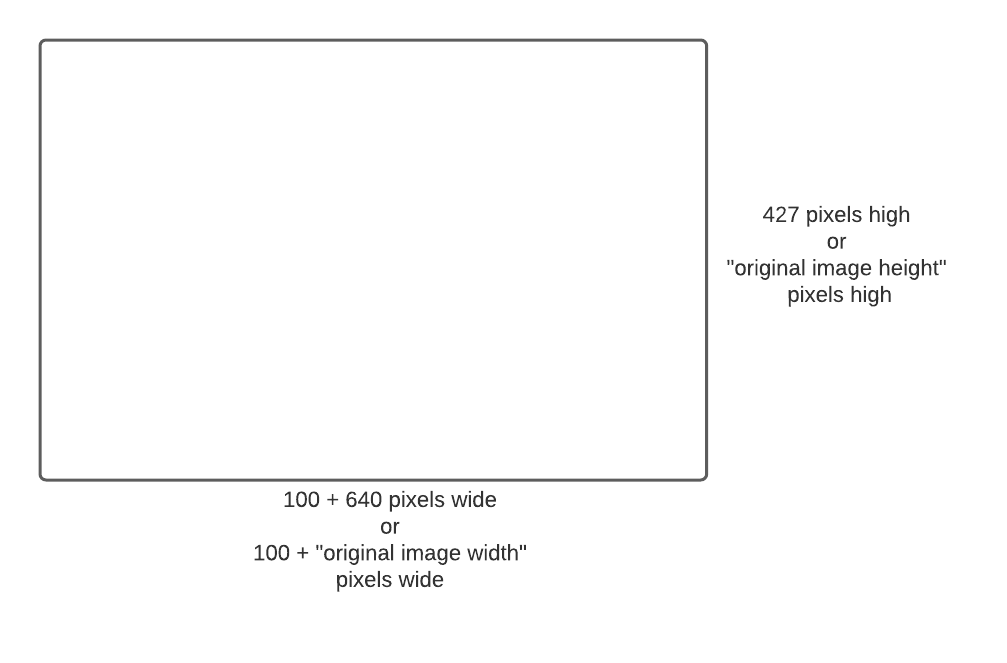### Planning — What do we need to do?

(2) Make an aqua stripe, 100 pixels wide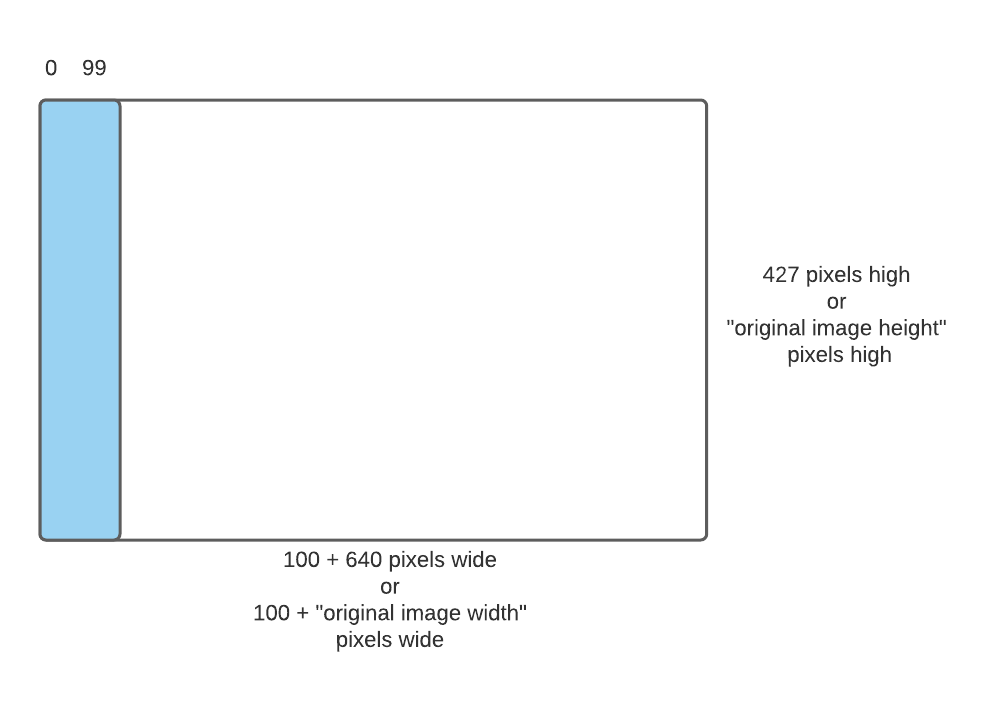### Planning — What do we need to do?

(3) Copy original image to right side of new image### Planning — What do we need to do?

original xoriginal yaqua xaqua y
001000
101010
201020
011001
111011
211021
• reduced image
• what is the relationship between original pixel and aqua pixel?
``````def aqua_stripe(original):
"""
Put an aqua stripe to the left of an image, 100 pixels wide
"""
border_width = 300
# Create a blank image 100 pixels wider than the original.
new_image = Image.blank(original.width + border_width, original.height)
# Put an aqua colored vertical stripe 100 pixels wide at the left by setting red to 0.
for y in range(new_image.height):
for x in range(border_width):
new_pixel = new_image.get_pixel(x, y)
new_pixel.red = 0
new_pixel.green = 0
new_pixel.blue = 0
# Copy the original image just to the right of the aqua stripe.
for y in range(original.height):
for x in range(original.width):
original_pixel = original.get_pixel(x, y)
new_pixel = new_image.get_pixel(x + 100, y)  # the key line
new_pixel.red = original_pixel.red
new_pixel.green = original_pixel.green
new_pixel.blue = original_pixel.blue
return new_image

original = Image("mount-timpanogos.jpeg")
aqua = aqua_stripe(original)
aqua.show()``````

### Can we generalize our solution?

• not a good idea to put the number 100 everywhere
• could easily have off-by-one errors
• see what happens if you use 101 or 99 in some places
• have to be sure we use “100” in 3 different places
• can we make a version that takes any width we specify?
``````def aqua_stripe(original, width):
"""
Put an aqua stripe to the left of an image
"""
# Create a blank image "width" pixels wider than the original.
new_image = Image.blank(original.width + width, original.height)
# Put an aqua colored vertical stripe at the left by setting red to 0.
for y in range(new_image.height):
for x in range(width):
new_pixel = new_image.get_pixel(x, y)
new_pixel.red = 0
# Copy the original image just to the right of the aqua stripe.
for y in range(original.height):
for x in range(original.width):
original_pixel = original.get_pixel(x, y)
new_pixel = new_image.get_pixel(x + width, y)  # the key line
new_pixel.red = original_pixel.red
new_pixel.green = original_pixel.green
new_pixel.blue = original_pixel.blue
return new_image

original = Image("mount-timpanogos.jpeg")
aqua = aqua_stripe(original,10)
aqua.show()``````

## Mirror Image Problem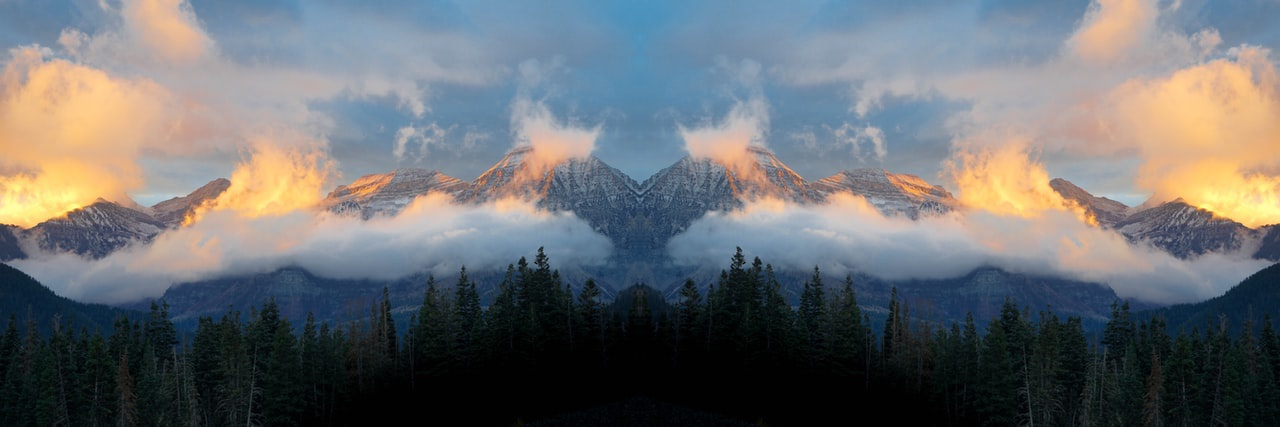### Planning

• What do you think the steps are?
• Class activity — talk to your neighbors

### Planning

• create a blank image that is twice as wide as the original
• copy original image to new image on the left half
• copy flip of original image to new image on the right half
• what is the formula for this?

### Planning

(1) create a blank image that is twice as wide as the original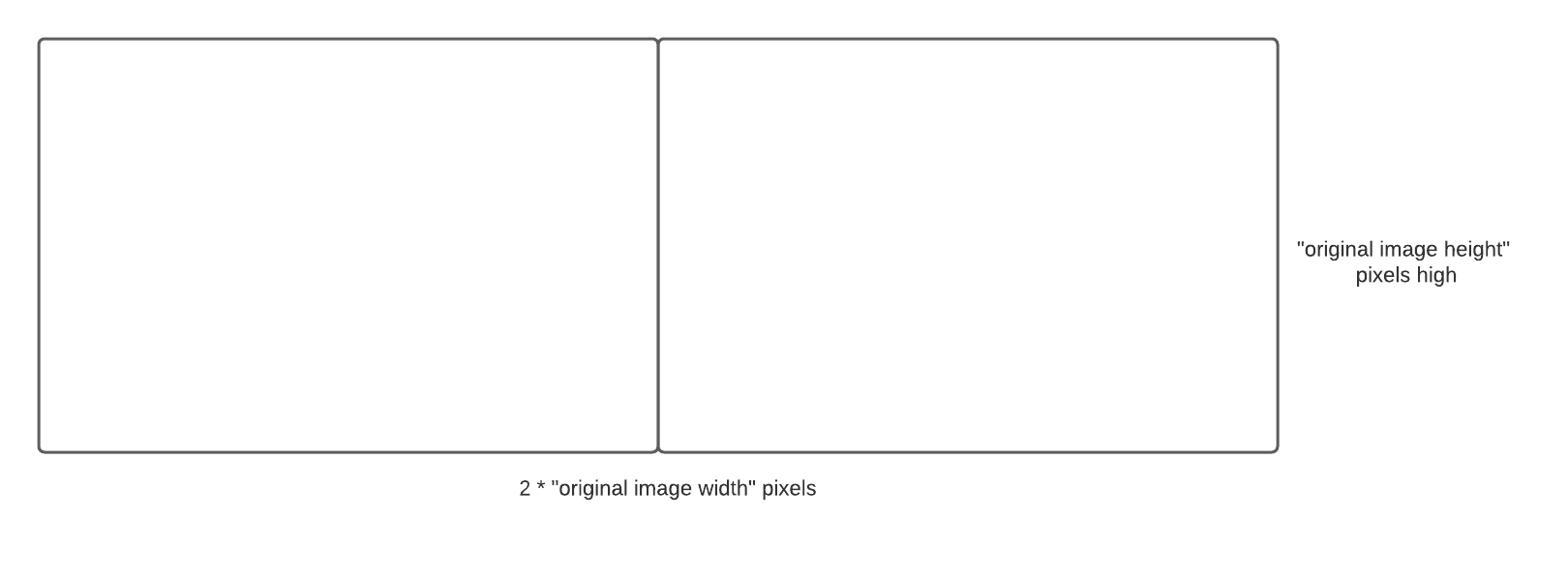### Planning

(2) copy original image to new image on the left half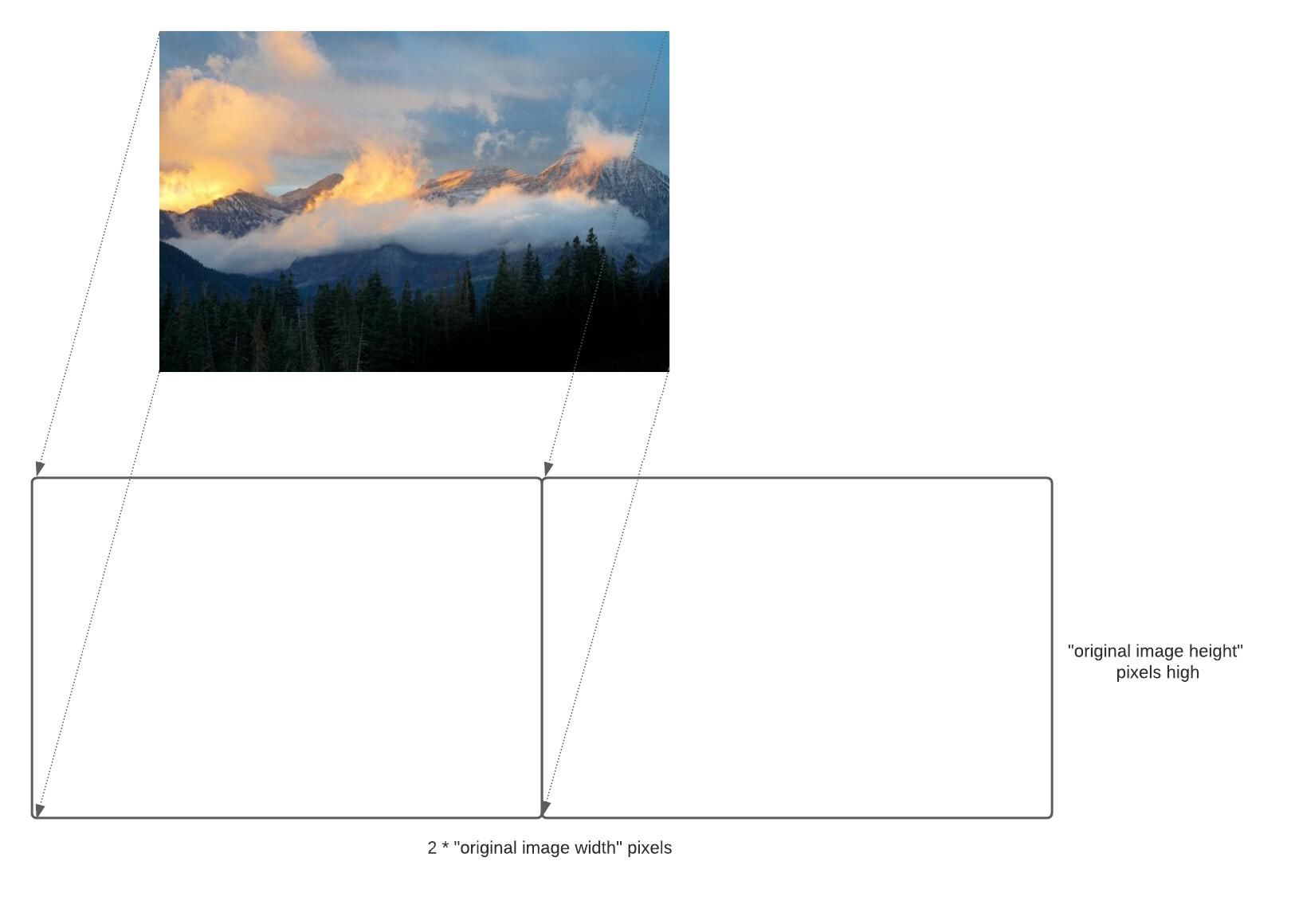### Planning

(3) copy flip of original image to new image on the right half### What is the formula?

• Pretend the original image is only 100 pixels wide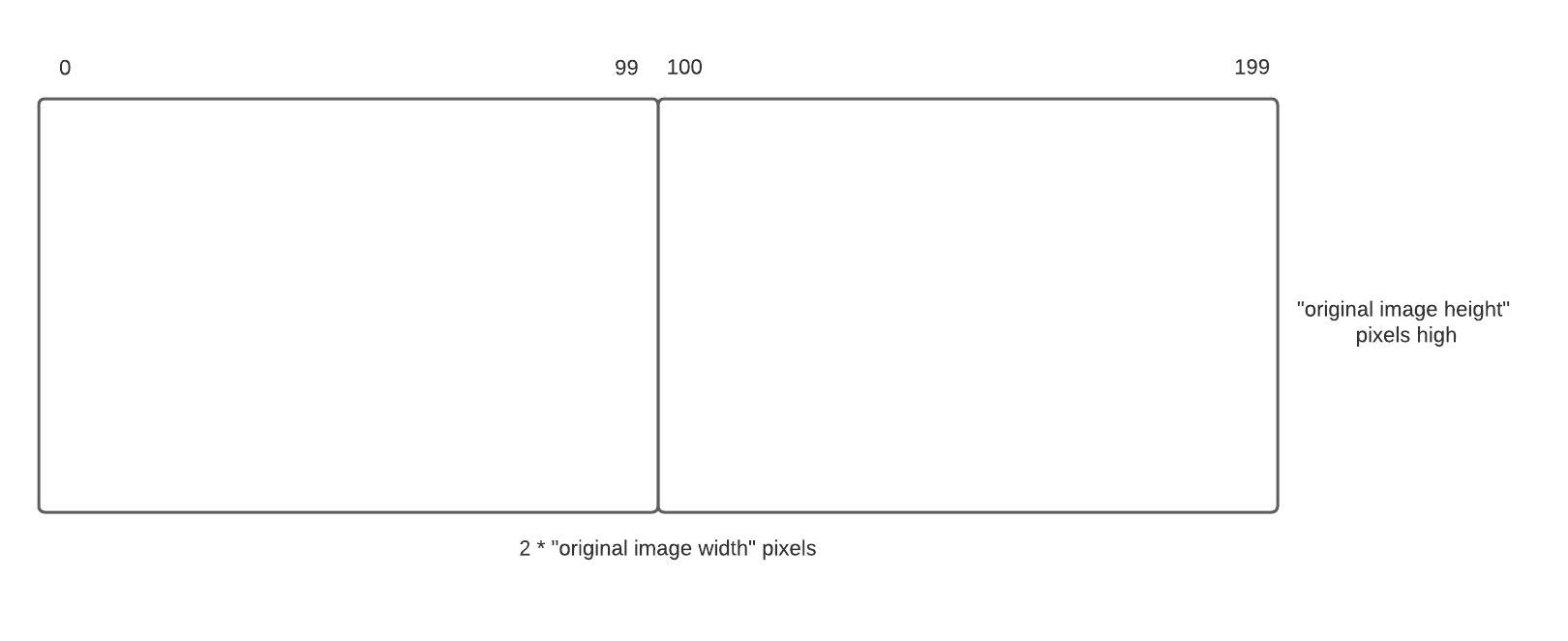• what are the equivalent coordinates in the new image? (talk to your neighbor)

• A: (0, 0), B: (1, 0), C: (2, 0), D: (99, 0)

### What is the formula?

original xoriginal ymirror xmirror y
001990
101980
201970
9901010

### What is the formula?

original xoriginal ymirror xmirror y
001990
101980
201970
9901010
• mirror_x = 200 - original_x - 1 = “new image width” - original_x - 1
• mirror_y = original_y
``````def create_blank_double_wide(original):
""" create a blank image that is twice as wide as the original

original: original image
returns: new image
"""
doubled = Image.blank(2 * original.width, original.height)
return doubled``````
``````def copy_left_half(original, doubled):
""" copy original image into left half of new image

original: original image
image: image to copy the original into
"""
for y in range(original.height):
for x in range(original.width):
pixel = original.get_pixel(x, y)
pixel_left = doubled.get_pixel(x, y)
pixel_left.red = pixel.red
pixel_left.green = pixel.green
pixel_left.blue = pixel.blue``````
``````def copy_right_half_mirror(original, doubled):
""" copy a mirror of the original image into right half of new image

original: original image
image: image to copy original into
"""
for y in range(original.height):
for x in range(original.width):
pixel = original.get_pixel(x, y)
pixel_right = image.get_pixel(doubled.width - 1 - x, y)
pixel_right.red = pixel.red
pixel_right.green = pixel.green
pixel_right.blue = pixel.blue``````
``````def mirror(original):
# create a blank image that is twice as wide as the original
doubled = create_blank_double_wide(original)
# copy original image to new image on the left half
copy_left_half(original, doubled)
# copy flip of original image to new image on the right half
copy_right_half_mirror(original, doubled)

return image

original = Image("mount-timpanogos.jpeg")
mirror_image = mirror(original)
mirror_image.show()``````